# Know How to Detect and Remove Loop in Linked ListA linked list is a computer science term that refers to a linear collection of data elements whose order is not provided by their physical placement in the memory. Rather, the elements are linked using pointers. This simply means that a linked list contains nodes where every single one comprises a data field and a reference link to the next node in the list. In this article, we will learn to detect and remove a loop in a linked list. This will be particularly helpful for data scientists as familiarization of a linked list as well as loops is important.

## Understanding loop in a linked list

A linked list that contains a loop will have the last node pointing to another node in the same list, rather than pointing to the NULL.

Removing a loop in a linked list means making the last node point to NULL and not to other nodes in the list.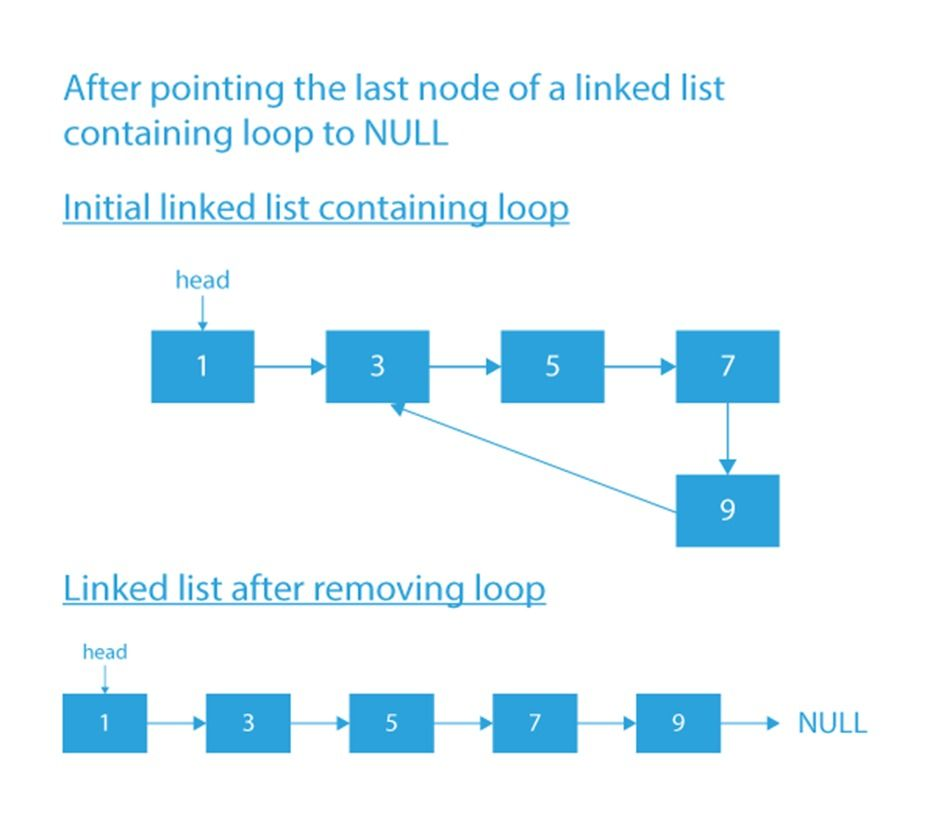Image source: PrepBytes

In the figure above, we can see that the linked list has a loop beginning at node 3 and contains 4 nodes, i.e., 3, 5, 7 and 9. We can also see that the last node redirects back to the first node.

Since we now know that the linked list has a loop in it, how do we go about removing it? Note that removing the loop will resemble something like this: 1 → 3 → 5 → 7 → 9 → NULL.

There are several ways we can remove a loop in a linked list. Let’s discuss this in detail

## How to find a loop in a linked list

### Approach 1: Floyd’s cycle-finding algorithm

The first step in diagnosing the problem is to detect if the linked list indeed has a loop. And the quickest way to do this is by using Floyd’s cycle-finding algorithm. It uses two pointers with one moving slower than the other as it traverses the list. The algorithm is also sometimes called the hare-tortoise algorithm as a reference to the children's story about the race between the hare and the tortoise.

In practice, Floyd's cycle-finding algorithm works as shown below:

Step 1: Both the pointers (ptr1 and ptr2) begin by pointing at the first node.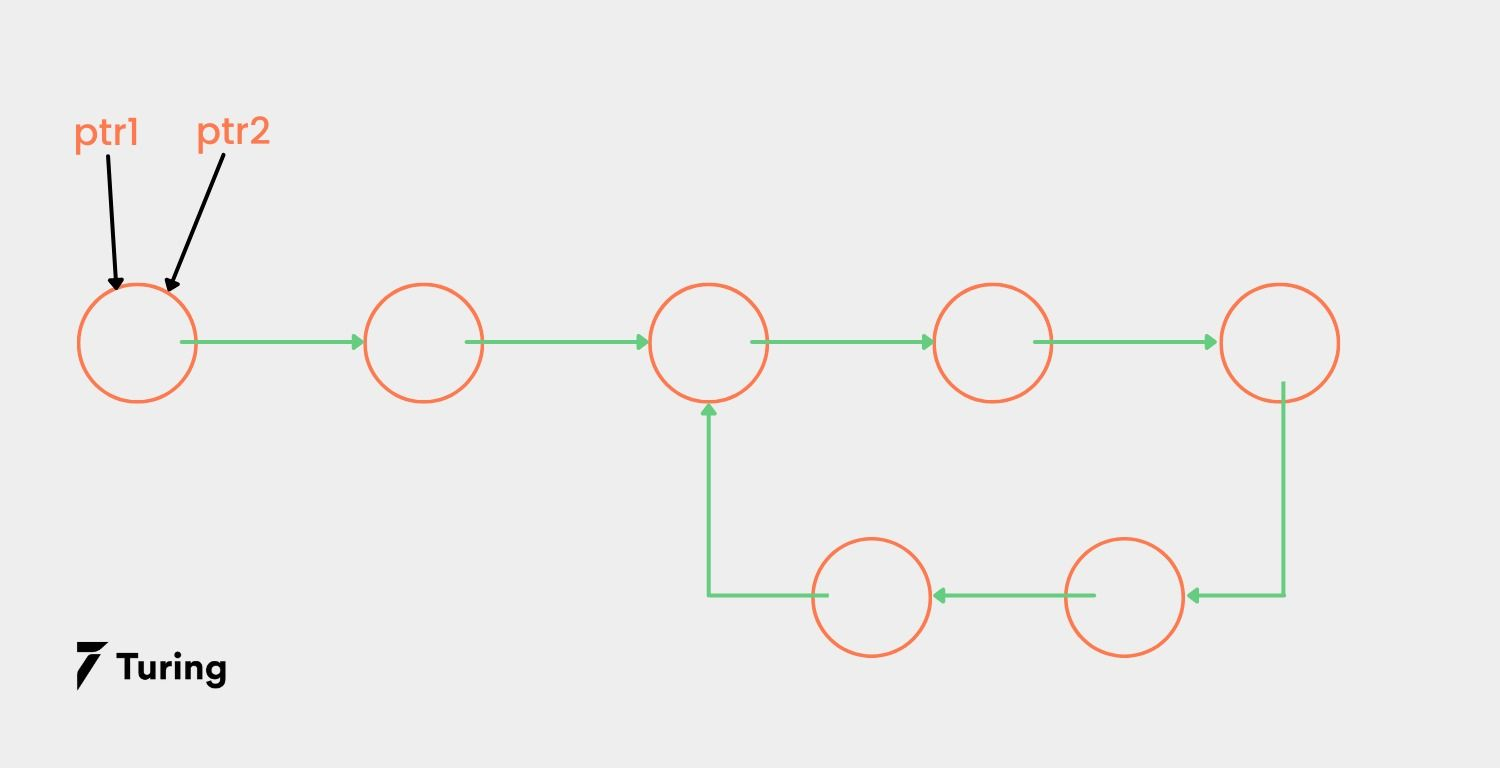Step 2: The two pointers start traversing the list, ptr1 one node at a time, and ptr2 two nodes at a time.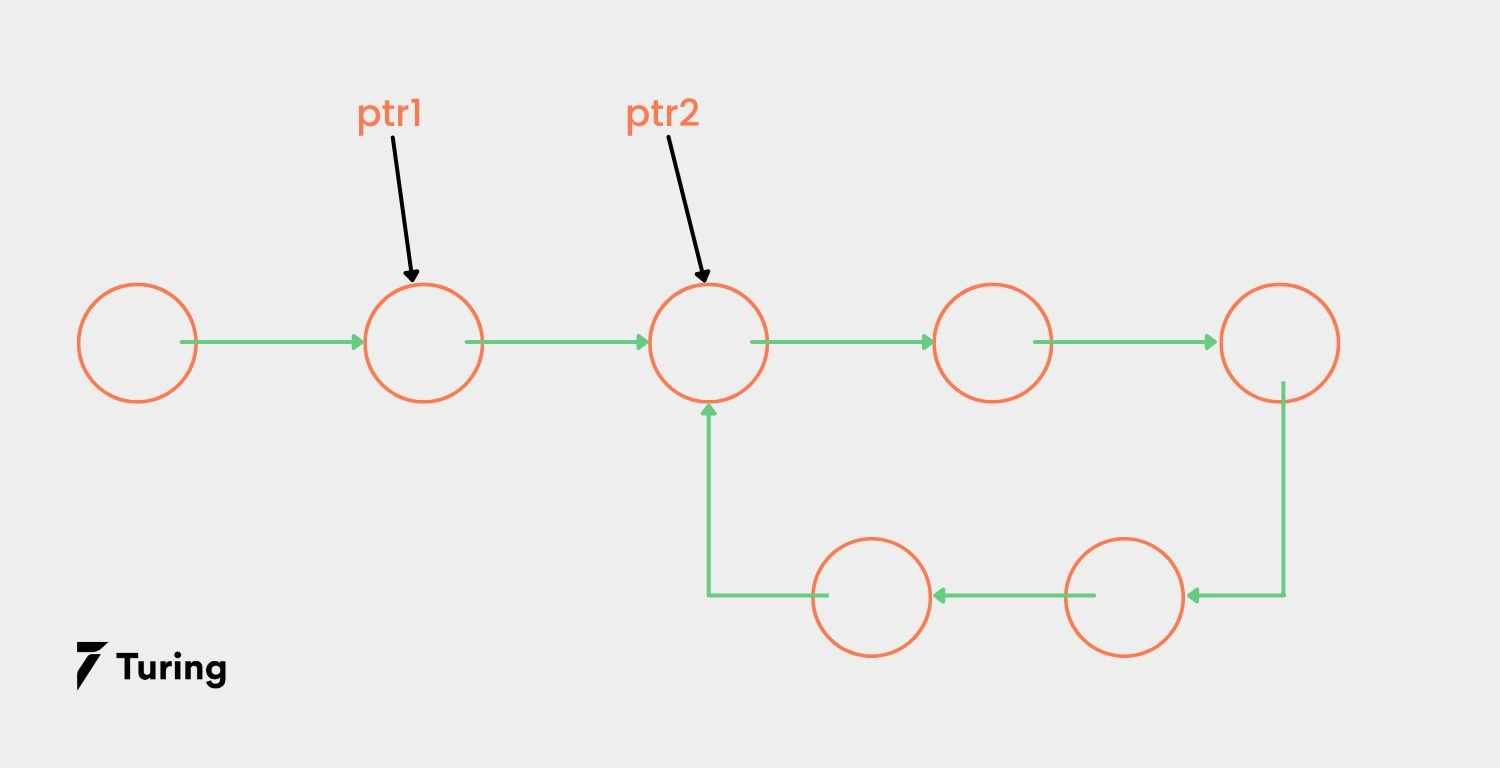Step 3: Since ptr2 moves faster than ptr1, it will reach the end of the linked list sooner.

After reaching the end of the list, there are two possible scenarios.

In the first scenario, there will be no loop in the list and therefore, ptr2→ will point to NULL since ptr2 moves two nodes at a go. This means that ptr2 will become null even if there are several nodes. Note that if there is an even number or an odd number of nodes in the linked list, ptr2→ will still become null.

In this case, a null value is encountered and we stop the execution and demonstrate the output of the code as ‘no loop’.

The second scenario is the presence of a loop in the linked list. As you may have already guessed, the pointers will not reach NULL. Instead, they will enter the loop and cross over there. Additionally, at some stage during traversing the list, the two pointers will eventually meet at a particular node.

Why is this? Because once the pointers find a loop, they will begin traversing in the loop with varying speeds, therefore ultimately meeting. When this happens, instead of finding a null value, it will be ptr1 = ptr2. Thus, the execution will stop and the code will show the output as ‘loop is present’.

Here are the images displaying the use of Floyd’s cycle-finding algorithm: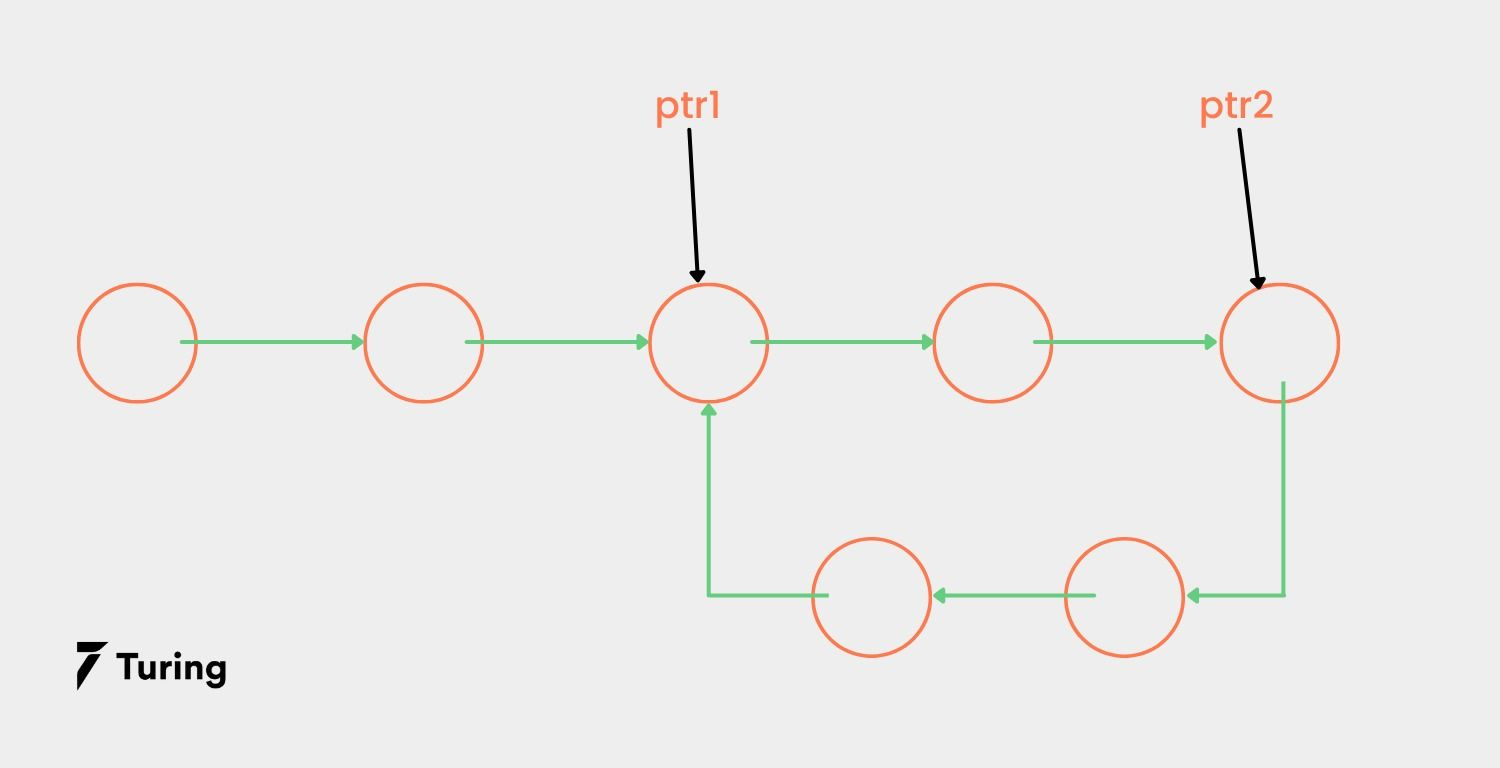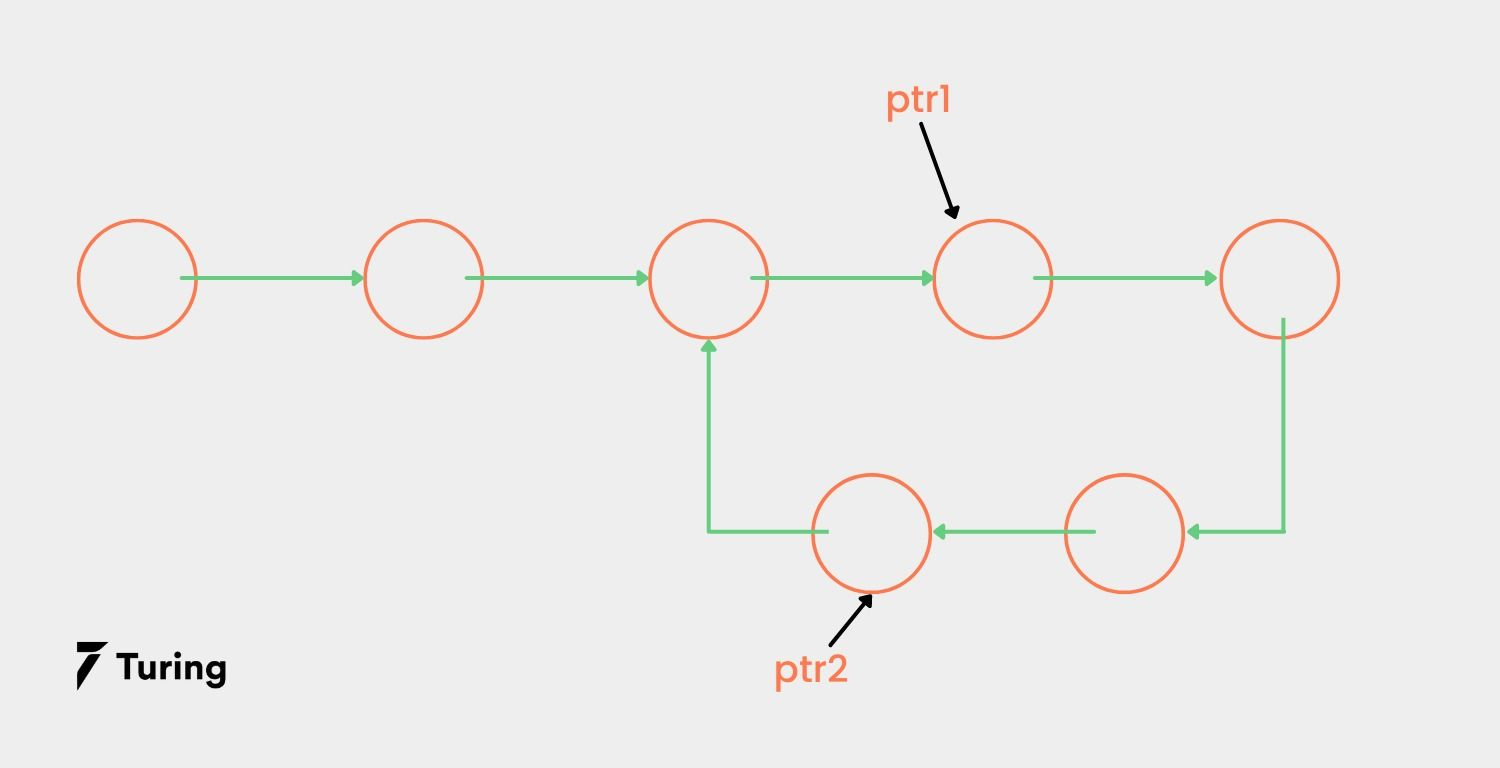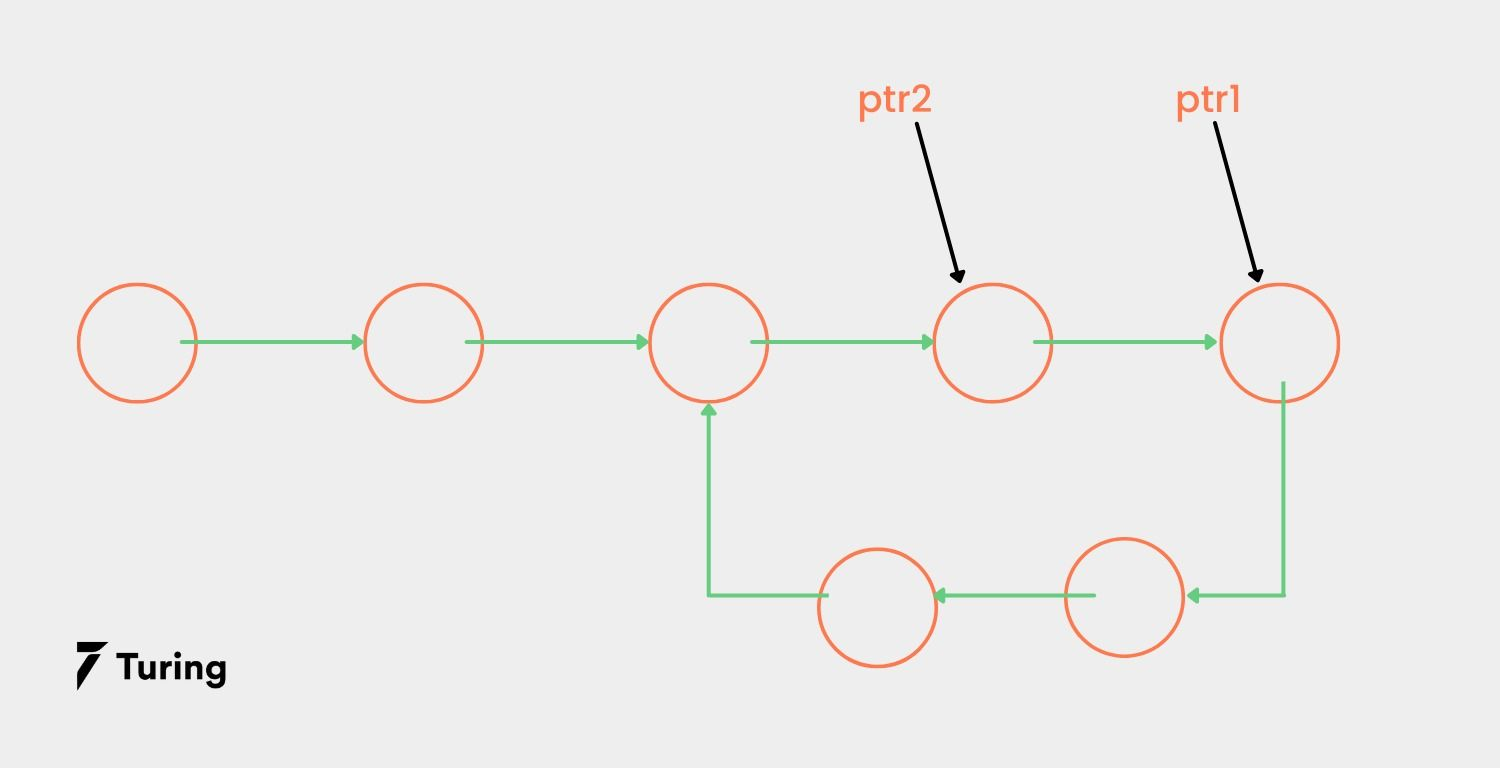As we can see, the two pointers will ultimately meet at a particular node in the loop.

Now, in the case of the first scenario, we stop there since there is no loop in the linked list. But in the case of the second scenario, we have to move to the second part of the problem – removing the loop.

#### How to remove a loop in a linked list

Let’s look at how we can remove the detected loop from our linked list so that the last element will point towards NULL. Here’s a step-by-step guide.

Step 1: As mentioned, ptr1 and ptr2 will finally point to the same node in the loop. So, we have to find the last element in the loop and make it point to NULL as shown below.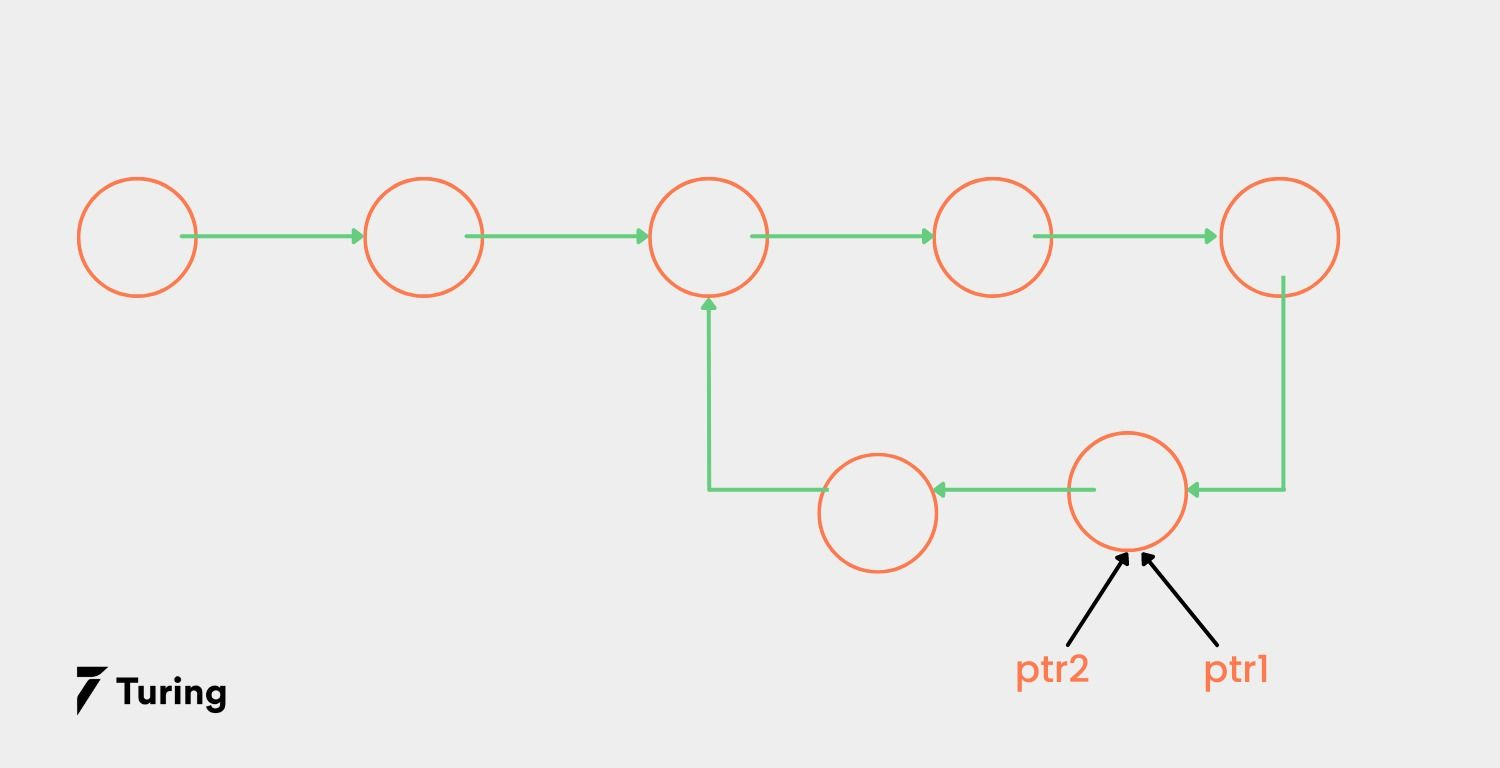Step 2: Leave ptr2 in its current location while making ptr1 point to the head of the loop as highlighted below.Step 3: Next, we traverse the two pointers through the linked list. Rather than ptr2 moving at a faster speed than ptr1, both pointers will move at the pace of ptr1, i.e., one node at a time. The traverse will continue until the two pointers eventually meet at one node. This is where the loop will begin.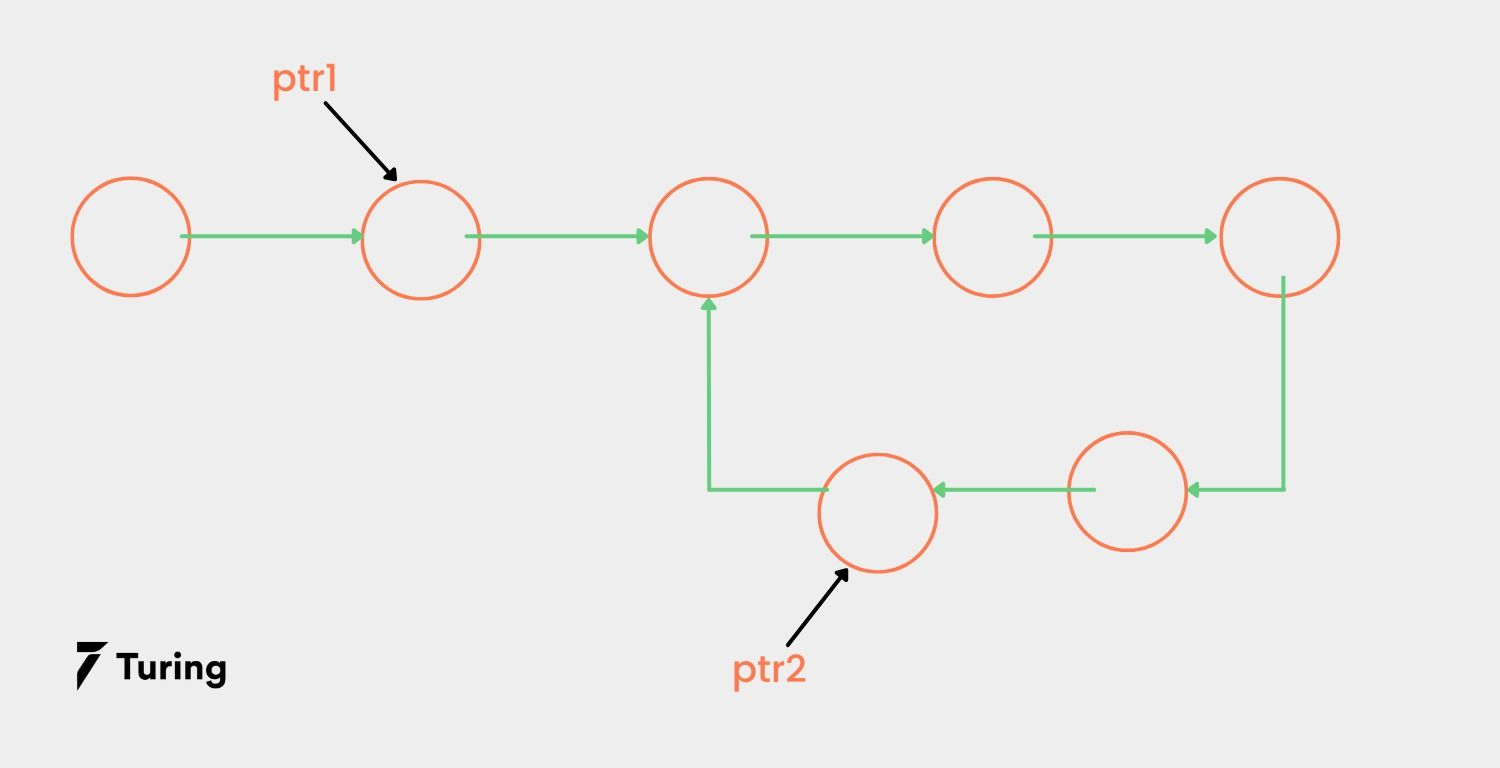Step 4: It’s time to remove the loop. We will make ptr2 point to the null value. Remember that the next of the two pointers will always meet at the start of the loop.

You may be wondering why the ‘next’ of ptr1 & ptr2 meets at the node that denotes the start of the loop. This is why:

Using this example,

L = number of nodes in the loop N = number of nodes in the list

So L = 5 and N = 12.

Starting with both pointers at the head node, ptr1 moves N-L steps or 7 steps for this example. Since ptr2 moves two nodes at a time or twice the speed of ptr1, ptr2 should move 2(N-L) steps or extra steps compared to ptr1.

This means the distance between the two pointers will be as (N-L) %L or in our case, (12-5)%5 = 2 nodes. Thus, ptr2 will move through the entire loop in the linked list to cover (N-L) nodes.

Next, the distance between the pointers will be (N-L)%L plus 1. In the next step after this, the distance will be (N-L)%L plus 2, (N-L)%L plus 3, and so on. This means that we can find the distance between the two points using the following formula (N-L)%L plus X, where ptr1 traverses X nodes from the beginning point of our loop.

However, the execution must be stopped the moment ptr1 and ptr2 meet at the same node. Now, the two will meet when the distance between them is L, since ptr2 will traverse the loop once and then meet with ptr1.

The distance between the two will be L itself when X = L – ( (N-L)%l). So, in our example above, X = 3.

Now, leaving ptr2 in its original location, we bring ptr1 to the head node. Both pointers will still move one node at a time, i.e., at the same speed. In this case, ptr1 moves N-L nodes to reach the node of the starting loop. Likewise, when ptr2 moves N-L nodes, it will reach the node of the starting loop, since ptr2 was 2 nodes from the initial point, i.e., (N-L)%L.

This means both ptr1 and ptr2 will always run into each other at the node of the starting loop. Therefore, to remove the loop from our linked list, we will go one step in reverse until ptr2 will be the node of the starting point of the loop, and then make the value of next ptr2, NULL.

Here is a code in C programming language for the problem:

``````#include<stdio.h>
#include<stdlib.h>

struct node{
int data;
struct node *next;
};

void removeLoop(struct node* head){

int flag =0;

//ptr1 and ptr2 initialized
struct node *ptr1 = head;
struct node *ptr2 = head;

while(ptr2!=NULL && ptr2->next!=NULL){
ptr1 = ptr1->next;
ptr2 = ptr2->next->next;

if(ptr1==ptr2){
//When a loop is present
flag=1;
break;
}
}

if(flag == 1){

while(ptr1->next != ptr2->next){
ptr1 = ptr1->next;
ptr2 = ptr2->next;
}

ptr2->next = NULL;
}
}

int main(){

struct node *head = (struct node*)malloc(sizeof(struct node));
struct node *one = (struct node*)malloc(sizeof(struct node));
struct node *two = (struct node*)malloc(sizeof(struct node));
struct node *three = (struct node*)malloc(sizeof(struct node));
struct node *four = (struct node*)malloc(sizeof(struct node));

head-> data = 21;
head-> next = one;

one-> data = 45;
one-> next = two;

two-> data = 3;
two-> next = three;

three-> data = 78;
three-> next = four;

four-> data = 10;
four-> next = two;

}
printf("NULL");
return 0;
}
``````

Output:

From the above code, the linked list with a loop is initialized first. We then applied Floyd’s algorithm to get rid of the loop and then print the output.

### Approach 2: Hashing

If you found the first method complicated, this next one is pretty straightforward. In this approach, we will use an unordered_map which will keep inserting nodes while moving through the list.

Once we meet a node that is in the map, it denotes that we have reached the node of the starting point of the loop.

While traversing the list, we ensure that the two pointers are at each step: head and last. The head points to the current node and the last node points to the preceding node.

At this juncture, the head is pointing to the starting point of the loop while the last is pointing to the preceding node of the node that the head was pointing to, i.e., pointing to the last node. To break the loop, we should make last -> next== NULL.

By doing this, the last node will point to NULL rather than to the starting node of our loop. The code for this problem is shown below in C++, Java, and Python languages

#### C++

``````#include <bits stdc++.h="">
using namespace std;

struct Node {
int key;
struct Node* next;
};

Node* newNode(int key)
{
Node* temp = new Node;
temp->key = key;
temp->next = NULL;
return temp;
}

{
while (head != NULL) {
cout << head->key << " ";
}
cout << endl;
}

{

unordered_map<node*, int=""> node_map;

Node* last = NULL;
while (head != NULL) {

if (node_map.find(head) == node_map.end()) {
}

else {
last->next = NULL;
break;
}
}
}

int main()
{
Node* head = newNode(1);

printf("After removing the loop we get : \n");

return 0;
}
</node*,></bits>
``````

#### Java

``````import java.util.*;
class DetectLoop {

static class Node {
int data;
Node next;
Node(int d)
{
data = d;
next = null;
}
}
static public void push(int new_data)
{

Node new_node = new Node(new_data);

}
void printList(Node node)
{
while (node != null) {
System.out.print(node.data + " ");
node = node.next;
}
}
static boolean removeLoop(Node h)
{
HashSet<node> s = new HashSet<node>();
Node prev = null;
while (h != null) {
if (s.contains(h)) {
prev.next = null;
return true;
}
else {
prev = h;
h = h.next;
}
}

return false;
}

public static void main(String[] args)
{
DetectLoop llist = new DetectLoop();

llist.push(20);
llist.push(4);
llist.push(15);
llist.push(10);

System.out.println("Linked List after removing loop");
}
else
System.out.println("No Loop found");
}
}

</node></node>
``````

#### Python

``````class Node:
def __init__(self, data, next=None):
self.data = data
self.next = next

while curr:
print(curr.data, end=' —> ')
curr = curr.next
print('None')

prev = None
s = set()
while curr:
if curr in s:
prev.next = None
return

prev = curr
curr = curr.next

if __name__ == '__main__':

``````

In this article, we learned how to get rid of loops in a linked list using extra pointers. We learned about Floyd’s cycle-finding algorithm to locate a loop within lists. To revamp, two pointers traversing the list at different speeds were used to find the node where the loop starts. We then calculated the exact node where the loop begins, and used the pointers to make the linked list linear by pointing the last node to NULL. We also explored a second method, hashing, to find the node where the loop begins and rid our list of loops.

As discussed, a linked list is one of the most important data types in computer programming. It is a collection of data elements that is accessed by iterating through the elements connected using pointers. Try the codes given above in your preferred programming language to detect if your linked list has loops and remove them.

## Author

•### Turing

Author is a seasoned writer with a reputation for crafting highly engaging, well-researched, and useful content that is widely read by many of today's skilled programmers and developers.

## Frequently Asked Questions

No, linked lists cannot be created using arrays. Arrays are continuous memory spaces for similar data type items, whereas linked lists are separate memory chunks connected using pointers.

A doubly linked list is immutable, but a normal singly linked list is mutable.

Both are popular data types in various programming languages. Arrays are pre-determined chunks of memory with immutable size, whereas linked lists are a collection of separate, ordered memory chunks connected using pointers.### Press

What's up with Turing? Get the latest news about us here.### Blog

Know more about remote work.
Checkout our blog here.### Contact

Have any questions?
We'd love to hear from you.

#### Hire and manage remote developers

Tell us the skills you need and we'll find the best developer for you in days, not weeks.